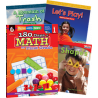•# Learn-at-Home Math Bundle Grade 1

Product Number: 9781425816896, EDM3996986
\$42.96
Quantity

#### Description

Give 1st grade students the confidence they need to approach a variety of math problems with this set that provides daily practice for every day of the school year. Featuring engaging math readers that illustrate how math concepts apply in real-world contexts, this math book set includes daily math worksheets to reinforce these key concepts.

This set includes 180 Days of Math for First Grade, an engaging math workbook that provides activities that cover the math concepts that students need to know to advance to the next level. The math activity sheets teach essential math skills including number sense, addition, subtraction, algebraic thinking, geometry, measurement, data analysis, word problems, logic problems, and mathematical reasoning. Titles include (math reader titles may vary):

• 180 Days of Math for First Grade
• A Mountain of Trash
• Let's Play!

#### Product Details

9781425816896, EDM3996986